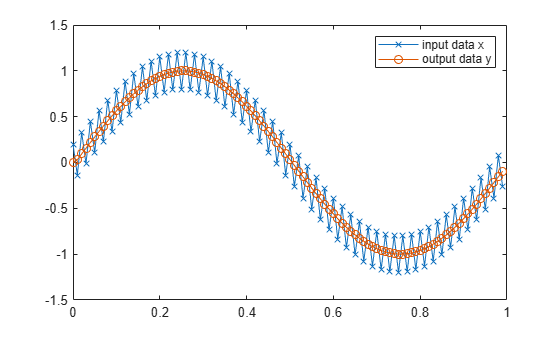subsasgn

Subscripted assignment

Description

example

A = subsasgn(A,S,B) is called for the syntax A(i) = B, A{i} = B, or A.i = B when A is an object.

MATLAB® uses the built-in subsasgn function to interpret indexed assignment statements:

• A(i) = B assigns the values of B into the elements of A specified by the subscript vector i. B must have the same number of elements as i or be a scalar value.

• A(i,j) = B assigns the values of B into the elements of the rectangular submatrix of A specified by the subscript vectors i and j. B must have length(i) rows and length(j) columns.

• A colon used as a subscript, as in A(i,:) = B or A(:,i) = B, indicates the entire column or row.

• For multidimensional arrays, A(i,j,k,…) = B assigns B to the specified elements of A. B must be length(i)-by-length(j)-by-length(k)-… or be shiftable to that size by adding or removing singleton dimensions.

Tip

You can use fixed-point assignment, for example, A(:) = B, to cast a value with one numeric type into another numeric type. This subscripted assignment statement assigns the value of B into A while keeping the numeric type of A. Subscripted assignment works the same way for integer data types.

Note

You must call subsasgn with an output argument. subsasgn does not modify the object used in the indexing operation (the first argument). You must assign the output to obtain a modified object.

Examples

collapse all

For fi objects a and b, there is a difference between

a = b

and

a(:) = b.

In the first case, a = b replaces a with b while a assumes the value, numeric type, and fimath object associated with b. In the second case, a(:) = b assigns the value of b into a while keeping the numeric type of a. You can use this to cast a value with one numerictype object into another numerictype object.

For example, cast a 16-bit number into an 8-bit number.

a = fi(0, 1, 8, 7)
a =
0

DataTypeMode: Fixed-point: binary point scaling
Signedness: Signed
WordLength: 8
FractionLength: 7
b = fi(pi/4, 1, 16, 15)
b =
0.7854

DataTypeMode: Fixed-point: binary point scaling
Signedness: Signed
WordLength: 16
FractionLength: 15
a(:) = b
a =
0.7891

DataTypeMode: Fixed-point: binary point scaling
Signedness: Signed
WordLength: 8
FractionLength: 7

Define the variable acc to emulate a 40-bit accumulator of a DSP. The products and sums in this example are assigned into the accumulator using the syntax acc(1)=.... Assigning values into the accumulator is like storing a value in a register. To begin, turn on the logging mode and define the variables. In this example, n is the number of points in the input data x and output data y, and t represents time. The remaining variables are all defined as fi objects. The input data x is a high-frequency sinusoid added to a low-frequency sinusoid.

fipref('LoggingMode', 'on');
n = 100;
t = (0:n-1)/n;
x = fi(sin(2*pi*t) + 0.2*cos(2*pi*50*t));
b = fi([.5 .5]);
y = zeros(size(x),'like',x);
acc = fi(0.0, true, 40, 30);

The following loop takes a running average of the input x using the coefficients in b . Notice that acc is assigned into acc(1)=... versus using acc=..., which would overwrite and change the data type of acc .

for k = 2:n
acc(1) = b(1)*x(k);
acc(1) = acc + b(2)*x(k-1);
y(k) = acc;
end

By averaging every other sample, the loop shown above passes the low-frequency sinusoid through and attenuates the high-frequency sinusoid.

plot(t,x,'x-',t,y,'o-')
legend('input data x','output data y')The log report shows the minimum and maximum logged values and ranges of the variables used. Because acc is assigned into rather than overwritten, these logs reflect the accumulated minimum and maximum values.

logreport(x, y, b, acc)
minlog         maxlog     lowerbound     upperbound     noverflows    nunderflows
x      -1.200012       1.197998             -2       1.999939              0              0
y     -0.9990234      0.9990234             -2       1.999939              0              0
b            0.5            0.5             -1      0.9999695              0              0
acc     -0.9990234      0.9989929           -512            512              0              0

Display acc to verify that its data type did not change.

acc
acc =
-0.0941

DataTypeMode: Fixed-point: binary point scaling
Signedness: Signed
WordLength: 40
FractionLength: 30

Reset the fipref object to restore its default values.

reset(fipref)

Input Arguments

collapse all

Object used in indexing operation, specified as a scalar, vector, or multidimensional array.

Data Types: single | double | int8 | int16 | int32 | int64 | uint8 | uint16 | uint32 | uint64 | logical | fi
Complex Number Support: Yes

Type of indexing and subscripts, specified as a structure array. S is a structure array with two fields:

• type is a character vector or string containing (), {}, or ., specifying the subscript type.

• subs is a cell array, character array, or string array containing the actual subscripts.

Example: The syntax A(1:2,:) = B calls a = subsasgn(A,S,B) where S is a 1-by-1 structure with S.type = '()' and S.subs = {1:2, ':'}. A colon used as a script is passed as ':'.

Data Types: struct

Value being assigned, specified as a scalar, vector, or multidimensional array.

Data Types: single | double | int8 | int16 | int32 | int64 | uint8 | uint16 | uint32 | uint64 | logical | fi
Complex Number Support: Yes

Output Arguments

collapse all

Result of assignment statement, which is the modified object passed in as the first argument, returned as a scalar, vector, or multidimensional array.

Extended Capabilities

GPU Code GenerationGenerate CUDA® code for NVIDIA® GPUs using GPU Coder™.